< >

# Neuigkeiten

erste Bilder von den
Skitagen in Disentis
Sedrun-Andermatt

|

die ersten Tests
sind online!

|

der Winter 19/20
startet bald!

|

alle neuen Anlagen hier

|

erste Tests der
Neuheiten 18/19

|

# Piecewise Cubic Bézier Curves

Written by Paul Bourke
March 2000

Given four points p0, p1, p2, and p3 in 3D space the cubic Bézier curve is defined as

p(t) = a t3 + b t2 + c t + p0

where t ranges from 0 (the start of the curve, p0) to 1 (the end of the curve, p3). The vectors a, b, c are given as follows:

c = 3 (p1 - p0)

b = 3 (p2 - p1) - c

a = p3 - p0 - c - b

In the following examples the green markers correspond to p0 and p3 of each section. The blue markers correspond to p1 and p2. The grey curve is the Bézier curve sampled 20 times, the samples are shown in red. The coordinates for each vertex is shown on the right.

Example 1
This is a single minimum piece of a piecewise Bézier curve. It is defined by 4 points, the curve passes through the two end points. The tangent at the end points is along the line to the middle two points.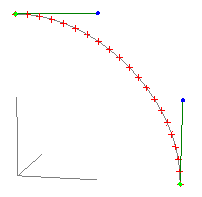0 0 1 0.5 0 1 1 0 0.5 1 0 0

Example 2
Multiple curve pieces can be joined together to form longer continuous curves. The curve is made continuous by the setting the tangents the same at the join. Note that each piece of the curve is defined by t ranging from 0 to 1.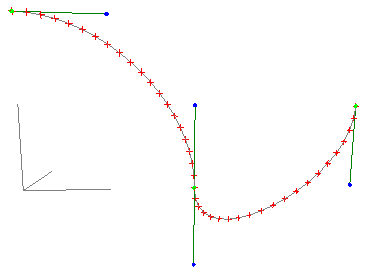0 0 1 0.5 0 1 1 0 0.5 1 0 0 1 0 0 1 0 -0.5 2 0 0 2 0 0.5

Example 3
By changing the tangent points between two curve pieces, sharp transitions can be created.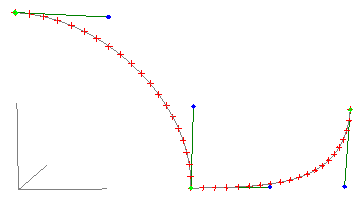0 0 1 0.5 0 1 1 0 0.5 1 0 0 1 0 0 1.5 0 0 2 0 0 2 0 0.5

Example 4
The "strength" at the end points is controlled by the length of the tangent lines. The longer the line the more effect that tangent has. If the curve is being used for animation steps then the strength also controls the velocity, note the samples shown in red are further apart for the long tangent vectors.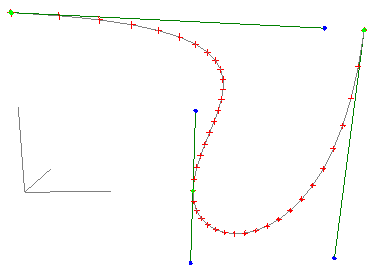0 0 1 1.75 0 1 1 0 0.5 1 0 0 1 0 0 1 0 -0.5 2 0 -0.5 2 0 1

Example 5
Straight line geometry can readily be made by aligning the tangent vectors along the line. While this may seem a frivolous use, it can be put to good effect in animations applications. By adjusting the tangent points p1 and p2 the velocity along the line can be controlled.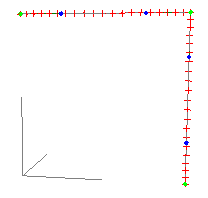0 0 1 0.25 0 1 0.75 0 1 1 0 1 1 0 1 1 0 0.75 1 0 0.25 1 0 0

Notes

• Piecewise cubic Bézier curves like their most general Bézier counterparts cannot exactly represent a circle.

• Except in the trivial case of a straight line, it isn't possible to create a Bézier curve that is parallel to another.

• There is no closed solution to finding the closest point on a Bézier curve to another point. The usual method is some kind of subdivision of t until some error tolerance is met.